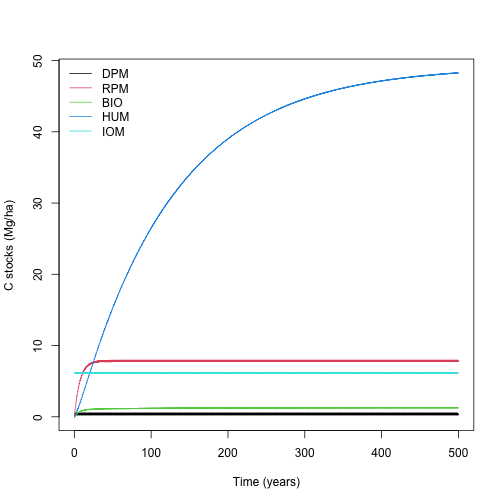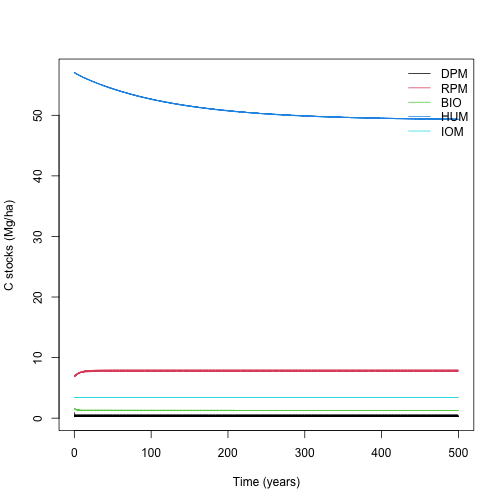RothC is a very popular model commonly used to explore soil carbon dynamics in natural and agricultural ecosystems. SoilR includes an implementation of the RothC model based on previous descriptions of this model. This implementation is fully described in the paper presenting SoilR version 1.0 (Sierra et al. 2012).

In this workflow we will present a series of examples about runing the RothC model for a site where a number of soil parameters are known and monthly climate data is available. The data from this example was provided by Christopher Poeplau and Martin Wiesmeier for an agricultural site in southern Bavaria, Germany.

We will consider three examples in this Workflow for running RothC: 1) without measured fractions and a spin-up run to bring the model to steady-state, 2) using the Zimmermann fractionation scheme (Zimmermann et al. 2007), and 3) using a the pedotransfer function (Falloon et al. 1998, Weihermuelller et al. 2013).

## 1. Using clay content, litter inputs, and climate data

The easiest way to run RothC for a site is assuming that the only information available are the percent clay content in the topsoil, the annual amount of litter inputs, and monthly averages of climatic variables. For this example, we have the following climate data

Temp=data.frame(Month=1:12, Temp=c(-0.4, 0.3, 4.2, 8.3, 13.0, 15.9,
18.0, 17.5, 13.4, 8.7, 3.9,  0.6))
Precip=data.frame(Month=1:12, Precip=c(49, 39, 44, 41, 61, 58, 71, 58, 51,
48, 50, 58))
Evp=data.frame(Month=1:12, Evp=c(12, 18, 35, 58, 82, 90, 97, 84, 54, 31,
14, 10))


We also have the following values for percent clay content and annual litter inputs as well as the thickness of the topsoil

soil.thick=25  #Soil thickness (organic layer topsoil), in cm
SOC=69.7       #Soil organic carbon in Mg/ha
clay=48        #Percent clay
Cinputs=2.7   #Annual C inputs to soil in Mg/ha/yr


We will run simulations for 50 years into the future using this information and the default parameterization in RothC. We create now a vector of time steps to run the simulation

years=seq(1/12,500,by=1/12)


The effects of climate on decomposition can be calculated now with the functions fT.RothC and fW.RothC as

fT=fT.RothC(Temp[,2]) #Temperature effects per month
fW=fW.RothC(P=(Precip[,2]), E=(Evp[,2]),
S.Thick = soil.thick, pClay = clay,
pE = 1.0, bare = FALSE)\$b #Moisture effects per month
xi.frame=data.frame(years,rep(fT*fW,length.out=length(years)))


Notice that these monthly environmental effects on decomposition are recycled over a period of 500 years.

Even though we will assume zero carbon as initial condition for all pools, we still need to know the size of the inert organic matter pool (IOM) because this pool do not change over time in RothC and it is independent on the model's dynamics. Falloon et al. (1998) proposed an empirical function to determine the size of this pool based on data of the total soil organic carbon stock. The function is expressed as

$IOM = 0.049 \cdot SOC^{1.139},$

therefore,

FallIOM=0.049*SOC^(1.139) #IOM using Falloon method


To run the model, we use the function RothCModel to initialize the model and create a SoilR Model object to which we will solve to calculate C stocks for each pool as

Model1=RothCModel(t=years,C0=c(DPM=0, RPM=0, BIO=0, HUM=0, IOM=FallIOM),
In=Cinputs, clay=clay, xi=xi.frame) #Loads the model
Ct1=getC(Model1) #Calculates stocks for each pool per month


The results can be plotted with the commands

matplot(years, Ct1, type="l", lty=1, col=1:5,
xlab="Time (years)", ylab="C stocks (Mg/ha)")
legend("topleft", c("DPM", "RPM", "BIO", "HUM", "IOM"),
lty=1, col=1:5, bty="n")The final size of the pools after this 500 year spinup are

poolSize1=as.numeric(tail(Ct1,1))
names(poolSize1)<-c("DPM", "RPM", "BIO", "HUM", "IOM")
poolSize1

##        DPM        RPM        BIO        HUM        IOM
##  0.3837275  7.8264810  1.2583828 48.2678506  6.1608157


## 2. Using data based on Zimmermann's fractionation scheme

Zimmermann et al. (2007) proposed a soil fractionation scheme to obtain the initial C fractions in a soil and run the RothC model. They proposed a combination of physical and chemical procedures to obtain the fractions: dissolved organic carbon (DOC), sand and stable aggregates (S+A), particulate organic matter (POM), silt and clay (s+c), and resistant organic carbon (rSOC). The values obtained for the soil in this example are:

DOC=1.9       #Dissolved organic carbon in Mg/ha
SA=4.7        #OM in sand plus aggregates in Mg/hg
POM=5.9       #Particulate organic matter in Mg/ha
sc=53.8        #OM in silt plus clay in Mg/ha
rSOC=3.4      #Recalcitrant soil organic carbon in Mg/ha


These soi fractions need to be transformed to the parameters required to run RothC. According to Zimmermann et al. (2007), we assume POM+DOC=DPM+RPM, with a splitting ratio of 0.112 (RPM is 88.8%, and DPM is 11.2% of POM+DOC) and SA+SC=BIO+HUM with a splitting ratio of 0.026 (HUM=97.4% of SC+SA and BIO=2.6%of SC+SA). Finally IOM is identical to rSOC.

DPMplusRPM=DOC+POM
RPM=0.888*(DPMplusRPM)
DPM=0.112*(DPMplusRPM)

BIOplusHUM=SA+sc
HUM=0.974*(BIOplusHUM)
BIO=0.026*(BIOplusHUM)
ZimmIOM=rSOC #IOM following Zimmermann's method


We can create now a new model with this initial pool sizes

Model2=RothCModel(t=years,C0=c(DPM, RPM, BIO, HUM, ZimmIOM),
Ct2=getC(Model2) #Calculates stocks for each pool per month


Plot the results

matplot(years, Ct2, type="l", lty=1, col=1:5,
xlab="Time (years)", ylab="C stocks (Mg/ha)")
legend("topright", c("DPM", "RPM", "BIO", "HUM", "IOM"),
lty=1, col=1:5, bty="n")and obtain the final pool sizes

poolSize2=as.numeric(tail(Ct2,1))
names(poolSize2)<-c("DPM", "RPM", "BIO", "HUM", "IOM")
poolSize2

##        DPM        RPM        BIO        HUM        IOM
##  0.3837275  7.8264810  1.2624904 49.3238610  3.4000000


## 3. Pedotransfer functions

Recently, Weihermueller et al. (2013) proposed a set of functions (pedotransfer functions) to obtain the initial values of RothC pool sizes using data on total carbon clay contents. Their functions are given by

RPMptf=(0.184*SOC + 0.1555)*(clay + 1.275)^(-0.1158)
HUMptf=(0.7148*SOC + 0.5069)*(clay + 0.3421)^(0.0184)
BIOptf=(0.014*SOC + 0.0075)*(clay + 8.8473)^(0.0567)


The DPM fraction is therefore calculated as the remainder of the sum of these fractions and the IOM calculated from the Falloon method as

DPMptf=SOC-FallIOM-RPMptf-HUMptf-BIOptf
c(DPMptf, RPMptf, BIOptf, HUMptf, FallIOM)

##  -0.014136  8.265680  1.236454 54.051186  6.160816


Notice that in this case the DPM fraction we obtained is negative, a result that is not realistic.

We can now run the model and plot the results nevertheless as an example

Model3=RothCModel(t=years,C0=c(DPMptf, RPMptf, BIOptf, HUMptf, FallIOM),
Ct3=getC(Model3) #Calculates stocks for each pool per month


Plot the results

matplot(years, Ct2, type="l", lty=1, col=1:5,
xlab="Time (years)", ylab="C stocks (Mg/ha)")
legend("topright", c("DPM", "RPM", "BIO", "HUM", "IOM"),
lty=1, col=1:5, bty="n")Again, we can calculate the final pool sizes and observe that the model converges to the same numbers as with the previous methods, even though we are starting the simulation with a negative number for the DPM pool.

poolSize3=as.numeric(tail(Ct3,1))
names(poolSize3)<-c("DPM", "RPM", "BIO", "HUM", "IOM")
poolSize3

##        DPM        RPM        BIO        HUM        IOM
##  0.3837275  7.8264810  1.2622870 49.2715658  6.1608157


## Take home message

You might have noticed that in all cases the model converges to the same final pool sizes. This is because this model, and actually all first-order pool models, converge to the same steady-state independent on the initial conditions. For a formal treatment of this topic see our rencent paper (Sierra and Mueller 2015).

If you want to use your independently calculated fractions and observe how the model behaves in the transient state towards the steady-state, it is useful to use these fractionation procedures. If you only want to run RothC starting from the steady-state pools, you don't need fractionation data, the model will go there independently where you start. You may save some time and money if you initialize the model with a value of zero for all pools except IOM. The IOM pool would need a to be initialized with either the function proposed by Falloon et al. (1998) or Zimmermann et al. (2007), because this pool does not change over time and remains constant with the value from the initialization.

## References

Falloon, P., Smith, P., Coleman, K., and Marshall, S. (1998). Estimating the size of the inert organic matter pool from total soil organic carbon content for use in the Rothamsted carbon model. Soil Biology and Biochemistry, 30:1207-1211.

Sierra, C.A., Mueller, M., and Trumbore, S.E. (2012). Models of soil organic matter decomposition: the SoilR package, version 1.0. Geosci. Model Dev., 5:1045-1060.

Sierra, C.A., and Mueller, M. (2015). A general mathematical framework for representing soil organic matter dynamics. Ecological Monographs, 85:505-524.

Weihermueller, L., Graf, A., Herbst, M., and Vereecken, H. (2013). Simple pedotransfer functions to initialize reactive carbon pools of the RothC model. European Journal of Soil Science, 64:567-575.

Zimmermann, M., Leifeld, J., Schmidt, M.W.I., Smith, P., and Fuhrer, J. (2007). Measured soil organic matter fractions can be related to pools in the RothC model. European Journal of Soil Science, 58:658-667.